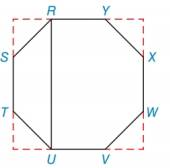Chapter 7.3, Problem 31EElementary Geometry For College St...

7th Edition
Alexander + 2 others
ISBN: 9781337614085

Solutions

Chapter
SectionElementary Geometry For College St...

7th Edition
Alexander + 2 others
ISBN: 9781337614085
Textbook Problem

Given regular octagon RSTUVWXY with each side of length 4, find the length of diagonal RU. (HINT: Extended sides, as shown, form a square.)To determine

To find:

The length of the diagonal RU.

Explanation

Consider the given figure.

Here, ABCD is a square with all vertex angles equal to 180°.

All four triangles are isosceles right triangle.

In triangle ASR, AS = AR

Use Pythagoras theorem in triangle ASR.

AR2+AS2=RS2AS2+AS2=422AS2=16AS2=8AS=22

In triangle DTU, DT = DU

Use Pythagoras theorem in triangle DTU.

DT2+DU2=TU2DT2+D

Still sussing out bartleby?

Check out a sample textbook solution.

See a sample solution

The Solution to Your Study Problems

Bartleby provides explanations to thousands of textbook problems written by our experts, many with advanced degrees!

Get Started

In Exercises 4756, solve the given equation for the indicated variable. 81=3x

Finite Mathematics and Applied Calculus (MindTap Course List)

Evaluate each expression: 83(92)217

Elementary Technical Mathematics

Differentiate the function. f(x) = x ln x x

Single Variable Calculus: Early Transcendentals, Volume I

In Exercises 2124, factor each expression completely. 23. 16 x2

Applied Calculus for the Managerial, Life, and Social Sciences: A Brief Approach

True or False: If h'(x) = k(x), then k(x) is an antiderivative of h(x).

Study Guide for Stewart's Single Variable Calculus: Early Transcendentals, 8th

The gradient vector field for is:

Study Guide for Stewart's Multivariable Calculus, 8th